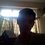# The factor problem

let us denote each number as the product of primes in a special way; for example
{2 3 5 7 11 ...}
{2 0 0 1 ...}
represents 28. (line up the primes in the first parentheses with the second one)
notice that in the prime factorization of 28, there a two 2's and one 7.
now, a further observation is that whenever we multiply two numbers, we add the number of each prime in that representation. for example 28 = [2 0 0 1] and 4 = [2 0 0 ...]. when we multiply them, we get 112 which is equal to [ 4 0 0 1]. similarly, when we raise a number to a power, we multiply each number in that "prime table" by that exponent. for example, 28^2 = [4 0 0 2], which is equal to 2x[2 0 0 1]. to summarize, multiplying numbers implies adding the corresponding prime tables and exponentiation of a number to a power implies multiplying every number in the prime table by the exponent.

now, the question is what addition of numbers translate to in the prime tables. all answers are appreciated.Note by Sri Prasanna
5 years, 5 months ago

This discussion board is a place to discuss our Daily Challenges and the math and science related to those challenges. Explanations are more than just a solution — they should explain the steps and thinking strategies that you used to obtain the solution. Comments should further the discussion of math and science.

When posting on Brilliant:

• Use the emojis to react to an explanation, whether you're congratulating a job well done , or just really confused .
• Ask specific questions about the challenge or the steps in somebody's explanation. Well-posed questions can add a lot to the discussion, but posting "I don't understand!" doesn't help anyone.
• Try to contribute something new to the discussion, whether it is an extension, generalization or other idea related to the challenge.
• Stay on topic — we're all here to learn more about math and science, not to hear about your favorite get-rich-quick scheme or current world events.

MarkdownAppears as
*italics* or _italics_ italics
**bold** or __bold__ bold
- bulleted- list
• bulleted
• list
1. numbered2. list
1. numbered
2. list
Note: you must add a full line of space before and after lists for them to show up correctly
paragraph 1paragraph 2

paragraph 1

paragraph 2

[example link](https://brilliant.org)example link
> This is a quote
This is a quote
    # I indented these lines
# 4 spaces, and now they show
# up as a code block.

print "hello world"
# I indented these lines
# 4 spaces, and now they show
# up as a code block.

print "hello world"
MathAppears as
Remember to wrap math in $$ ... $$ or $ ... $ to ensure proper formatting.
2 \times 3 $2 \times 3$
2^{34} $2^{34}$
a_{i-1} $a_{i-1}$
\frac{2}{3} $\frac{2}{3}$
\sqrt{2} $\sqrt{2}$
\sum_{i=1}^3 $\sum_{i=1}^3$
\sin \theta $\sin \theta$
\boxed{123} $\boxed{123}$

## Comments

There are no comments in this discussion.

×

Problem Loading...

Note Loading...

Set Loading...